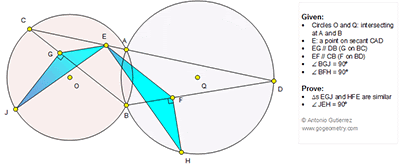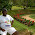## Tuesday, July 14, 2015

### Geometry Problem 1135: Intersecting Circles, Secant, Parallel Line, 90 Degrees, Similar Triangles

Level: Mathematics Education, High School, Honors Geometry, College.

Click the diagram below to enlarge it.1.http://s13.postimg.org/7538xe84n/Pro1135.png

Observe that following triangles are similar : CBD, CGE, EFD and OBQ ( case AA)
And triangle COB similar to BQD ( case SSS)
We have following ratios:
BC/BD=BO/BQ=GC/GE=EF/FD=BG/FD=GC/BF=OM’/QM= ON’/QN=OJ/OL= k
And triangle ON’J similar to QNL( similar of right triangles) => JN’/LN=JG/LF= k
From above result will lead to JG/GB=JG/EF= LF/FD=BF/FH=GE/FH
So trinagles EGJ and HFE are similar ( case SAS)

2.Peter Tran,

I don't understand this ON'/ QN= OJ/OL= k
How did you come in this result.

Thank you

1.Observe that
M’C/MB=k=GC/BF=(M’C-GC)/(MB-BF)=M’G/MF=ON’/QN=k

Peter Tran

3.HI Peter,

Why angle JEH=90 degree?

Thank You

1.Observe that ON’J similar to tri. QNL
So JN’/NL= GN’/NF= k= (JN’+GN’)/(NL+NF)=GJ/LF= k
Similarly GB/FD= k => GJ/LF=GB/FD
Note that ∠ (GEF)= ∠ (EFD) and ∠ (EFL)= ∠ (FHE)+ ∠ (FEH)
But ∠ (GEF)- ∠ (EFL)= ∠ (LFD)=90
From above we can get ∠ (JEH)=90

Peter Tran

4.Name M intersecting of circle Q with HF.
Connect point J with C and B
point M with B and D

1. CG/GB=BF/FD (=CE/ED)=BC/BD
2. ∠OBQ= ∠QBD => ∠ OBC= ∠ BQD=> ∠ CJB= ∠BMD
3. Δ BCJ~ Δ BMD ( CG/GB=BF/FD =BC/BD; ∠BJC=∠BMD; JG⊥BC; FM⊥BD)
MF/FD=JG/GB MF/FD=BF/FH JG/EF=GE/FH => Δ JGE~ ΔEFH ( case SAS)
4. ∠GEF=∠EFD ∠EFM=∠FEH+∠JGE
∠JEH=∠QEF- (∠GEJ+∠HEF)= ∠EFD-∠EFM=90º

5.Let JG = u, FH = v, CG = w, GE = x = BF, BG = y, EF

u2 = wy ….(1)

v2 = xz …. (2)

w/x = y/z ….(3) since Tr.s CGE and EFD are similar

So (u2 / y) / x = y /( v2 / x) from which,
u/x = y/v and since < JGE = < EFH,
Tr.s JGE & EFH are similar

Now
< JEH = < GEF - < GEJ - < HEF
= < GEF - < GEJ - < GJE
= 180 - < EGB - < GEJ - < GJE
= 90 considering Tr. JGE

Sumith Peiris
Moratuwa
Sri Lanka